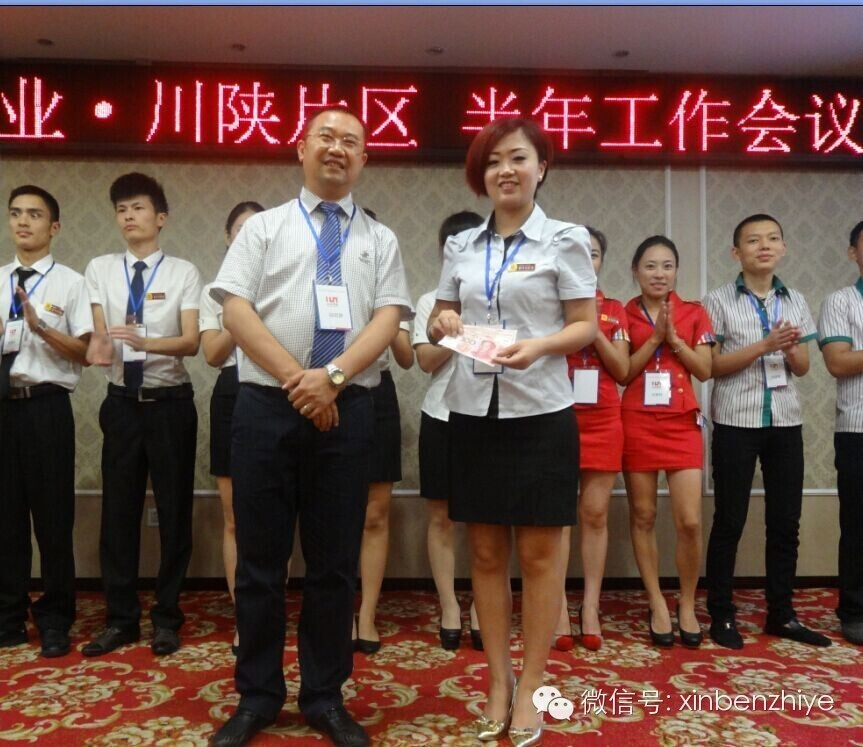theme->baseUrl; ?>/js/jquery.js"> theme->baseUrl; ?>/js/lrtk.js"> \$(document).ready(function () { \$(".rslides1_s1").html('素食珍馐'); \$(".rslides1_s2").html('文化足浴'); \$(".rslides1_s3").html('主题酒店'); \$(".rslides1_s4").html('智能软件'); \$(".rslides1_s5").html('树德公益'); });## 心本智业川陕片区半年度工作会议圆满召开2014年9月5日，心本智业川陕片区半年度工作总结会议在成都温江拉菲国际酒店顺利召开。来自四川及陕西片区的各分店管理人员及员工代表齐聚一堂，畅谈共举。管理公司执行总裁邱启彦及部分股东代表受邀参加会议，会议由片区总经理周显龙主持。
本次会议认真总结上半年度的经营状况，分析当前面临的市场形势，要求各分店夯实管理基础，提升服务品质，为下半年度各项工作的开展奠定基础。

\$(function(){ var slide = \$('.slideplay'), ulShow = \$('.slideshow'), sLi = ulShow.find('li'), bLi = \$('.loadbar li'), len = sLi.length; var option ={ speed:3000, barWidth:142 }, barSpeed = option.speed-100; var w = sLi.first().width(), h = sLi.first().height(); var flag = 0, timer = null; ulShow.css({ width:w+'px', height:h+'px' }); slide.css({ width:w+'px', height:h+'px' }); init(); function init(){ //set slide width and height by image sLi.eq(flag).addClass('zindex').show(); bLi.eq(flag).find('em').animate({width:option.barWidth},barSpeed); timer = setTimeout(function(){ next(); },option.speed); ulShow.on('mouseover',doStop); ulShow.on('mouseleave',doAuto); bLi.on('mouseover',handPattern); bLi.on('mouseout',handOut); } function handPattern(){ doStop(); flag = \$(this).index(); imgMove(); for(i=0;i<=flag;i++) {bLi.eq(i).find('em').width(option.barWidth);} for(i=flag+1;i<=len-1;i++) {bLi.eq(i).find('em').width(0);} //bLi.find('em').css('width',0); bLi.eq(flag).find('em').width(option.barWidth); } function handOut(){ timer = setTimeout(function(){ if(flag+1 == len) {bLi.eq(flag).find('em').css('width',0);} next(); },option.speed); } function doStop(){ timer && clearTimeout(timer); bLi.eq(flag).find('em').stop(); } function doAuto(){ var em = bLi.eq(flag).find('em'), w = em.width(), leftW = option.barWidth - w , sec = (leftW * barSpeed)/option.barWidth; em.animate({width:option.barWidth},sec,function(){ if(flag==len-1){ em.width(0); next(); }else{ next(); } }); } function next(){ flag++; if(flag==len) bLi.find('em').css('width',0); flag==len && (flag=0); /*for(i=0;i<=flag;i++) {bLi.eq(i).find('em').width(option.barWidth);} for(i=flag+1;i<=len-1;i++) {bLi.eq(i).find('em').width(0);} */ doMove(); } function doMove(){ imgMove(); loadbarMove(); } function imgMove(){ sLi.eq(flag).addClass('zindex').fadeIn('slow') .siblings().removeClass('zindex').fadeOut('slow'); } function loadbarMove(){ bLi.eq(flag).find('em'). animate({width:option.barWidth},barSpeed,function(){ if(flag==len-1){ bLi.find('em').width(0); next(); }else{ next(); } }); } })